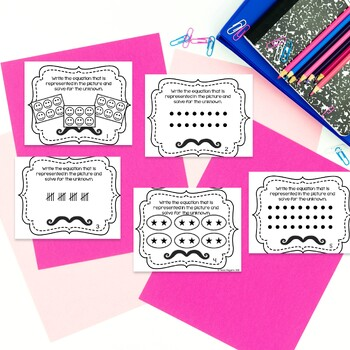# Multiplication & Division Task Cards BundleSubject
Resource Type
File Type

Zip

(4 MB|81 pages)
Product Rating
Standards
• Product Description
• StandardsNEW
Multiplication and Division Task Cards Bundle

Save 25% by purchasing the bundle!

This Mustache You the Equation Multiplication and Division Task Cards Bundle gives students practice on recognizing and applying multiplication and division skills. Students build conceptual understanding of multiplication and division with number lines, arrays, pictures of equal groups, and scenario tables. The bundle also includes practice on the distributive property and facts to 12.

This bundle includes 10 sets of task cards (each set has 28+ cards in it) with a mustache theme as well as a recording sheet. That gives you 296 task cards!

Answer keys and recording sheets are included with each file!

********************************************

The Multiplication and Division products included:
Multiplication & Division on a Number Line
Multiplication & Division Picture & Array Representations
Multiplication & Division Scenario Tables
Distributive Property of Multiplication
Multiplication & Division Facts 0 to 12

Click each set above to see each product.

********************************************

For other products practicing multiplication and division check out:
Multiplication & Division Story Problem Task Cards
Multiplication Wheels
Division Wheels

For other 3rd grade math products check out:
3rd Grade Problem of the Day Story Problems Bundle
3rd Grade CCSS Daily Math Warm Ups
Interpret a multiplication equation as a comparison, e.g., interpret 35 = 5 × 7 as a statement that 35 is 5 times as many as 7 and 7 times as many as 5. Represent verbal statements of multiplicative comparisons as multiplication equations.
Fluently multiply and divide within 100, using strategies such as the relationship between multiplication and division (e.g., knowing that 8 × 5 = 40, one knows 40 ÷ 5 = 8) or properties of operations. By the end of Grade 3, know from memory all products of two one-digit numbers.
Understand division as an unknown-factor problem. For example, find 32 ÷ 8 by finding the number that makes 32 when multiplied by 8.
Apply properties of operations as strategies to multiply and divide. Examples: If 6 × 4 = 24 is known, then 4 × 6 = 24 is also known. (Commutative property of multiplication.) 3 × 5 × 2 can be found by 3 × 5 = 15, then 15 × 2 = 30, or by 5 × 2 = 10, then 3 × 10 = 30. (Associative property of multiplication.) Knowing that 8 × 5 = 40 and 8 × 2 = 16, one can find 8 × 7 as 8 × (5 + 2) = (8 × 5) + (8 × 2) = 40 + 16 = 56. (Distributive property.)
Determine the unknown whole number in a multiplication or division equation relating three whole numbers. For example, determine the unknown number that makes the equation true in each of the equations 8 × ? = 48, 5 = __ ÷ 3, 6 × 6 = ?.
Total Pages
81 pages
Included
Teaching Duration
N/A
Report this Resource to TpT
Reported resources will be reviewed by our team. Report this resource to let us know if this resource violates TpT’s content guidelines.\$12.00
List Price:
\$16.00
You Save:
\$4.00